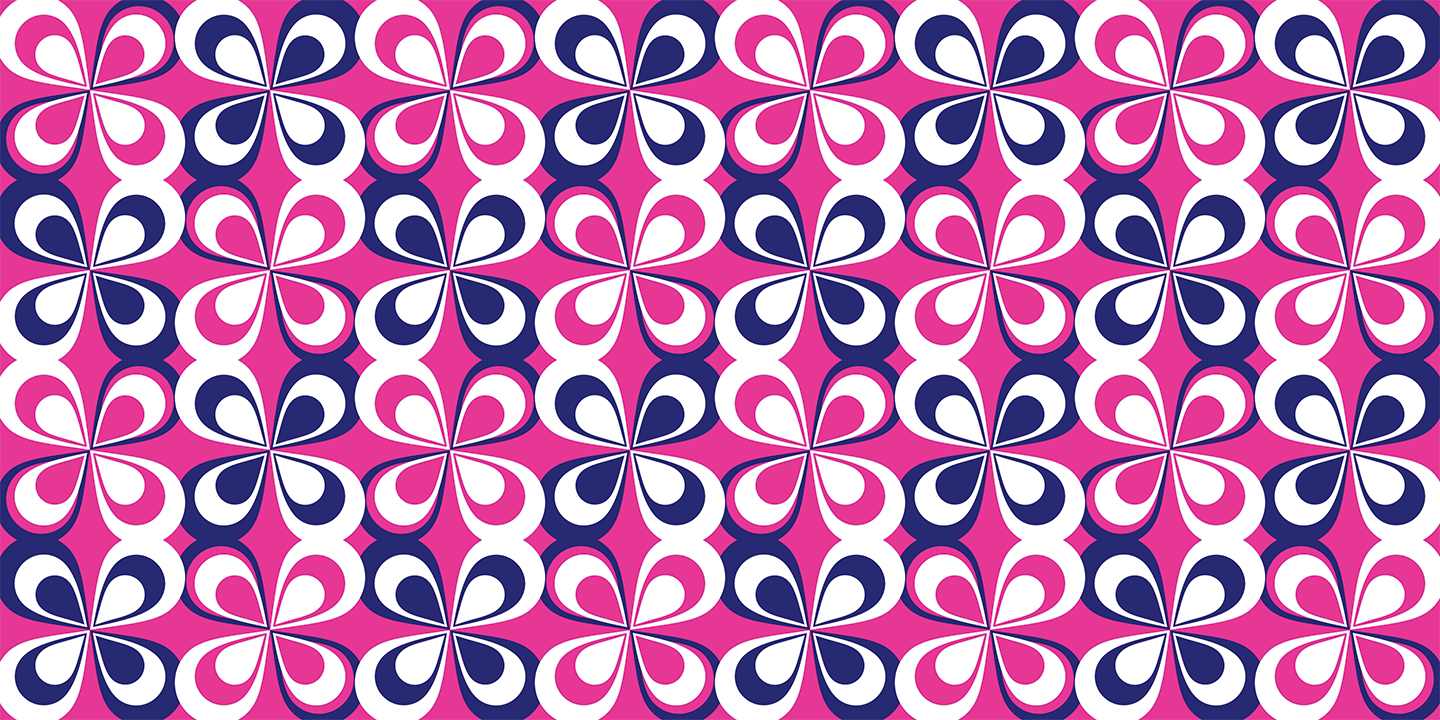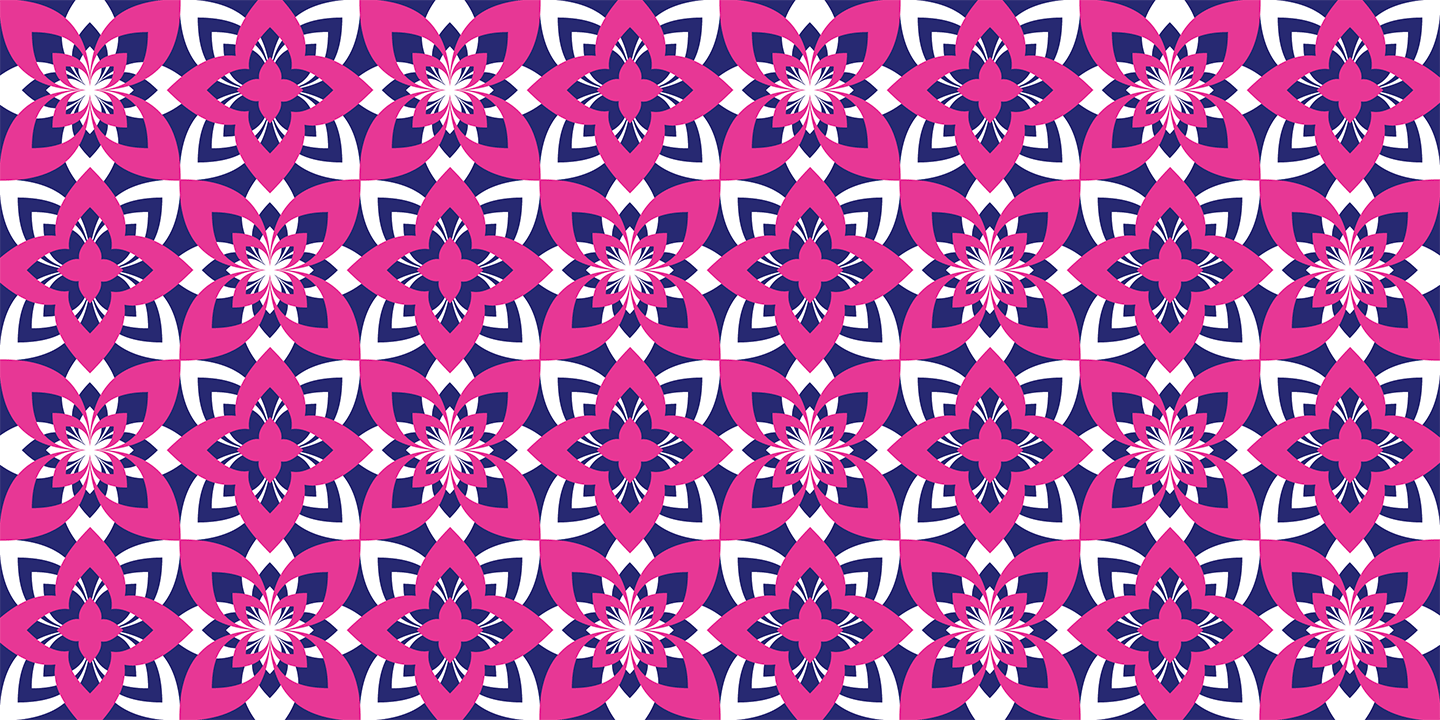# Puzzler

Designed by Zuzana Licko in 2005. More...

With Puzzler, Zuzana Licko revisits and expands upon some of her earlier forays into decoration and geometric constructions of abstract elements.

Licko was always intrigued by particular patterns such as the abstract dot compositions of blown-up halftone photographs, the interference patterns of superimposed grids known as moire, and the shadows of tree leaves on the sidewalk. These were some of the inspirations for the Puzzler patterns.

Some Puzzler elements started as dot grids of various sizes and frequencies, while others started as leaf or floral shapes that were arranged into interlocking compositions. In fact, many came about as happy accidents–some elements looked too random or uninteresting until their repetitions surprised her with unexpectedly fluid patterns.

The elements are arranged into three categories of fonts: Puzzler Halftone, Puzzler Geometric and Puzzler Floral. Each element is accessed through a letter on the keyboard, making it easy to repeat elements with precise scaling and positioning.

A
A

## Puzzler (268 Pattern Elements) \$115.00

A a B b C c D d E e F f G g H h I i J j K k L l M m N n O o P p Q q R r S s T t U u V v W w X x Y y Z z

## Puzzler Floral

A a B b C c D d E e F f G g H h I i J j K k L l M m N n O o P p Q q R r S s T t U u V v W w X x Y y Z z

## Puzzler Geometric

A a B b C c D d E e F f G g H h I i J j K k L l M m N n O o P p Q q R r S s T t U u V v W w X x Y y Z z

## Puzzler Halftone Coarse

A a B b C c D d E e F f G g H h I i J j K k L l M m N n O o P p Q q R r S s T t U u V v W w X x Y y Z z